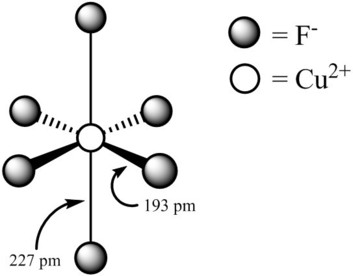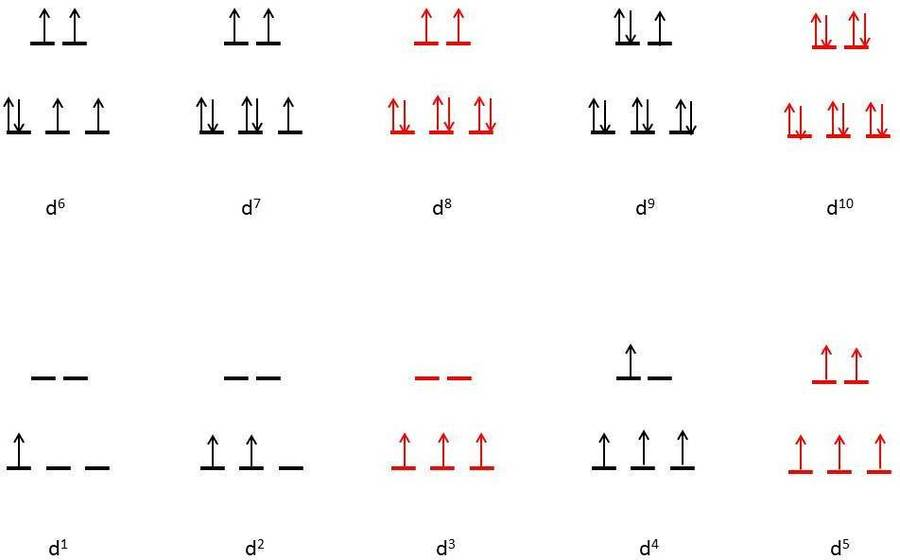# 1.04: Jahn-Teller Distortions

$$\newcommand{\vecs}{\overset { \rightharpoonup} {\mathbf{#1}} }$$ $$\newcommand{\vecd}{\overset{-\!-\!\rightharpoonup}{\vphantom{a}\smash {#1}}}$$$$\newcommand{\id}{\mathrm{id}}$$ $$\newcommand{\Span}{\mathrm{span}}$$ $$\newcommand{\kernel}{\mathrm{null}\,}$$ $$\newcommand{\range}{\mathrm{range}\,}$$ $$\newcommand{\RealPart}{\mathrm{Re}}$$ $$\newcommand{\ImaginaryPart}{\mathrm{Im}}$$ $$\newcommand{\Argument}{\mathrm{Arg}}$$ $$\newcommand{\norm}{\| #1 \|}$$ $$\newcommand{\inner}{\langle #1, #2 \rangle}$$ $$\newcommand{\Span}{\mathrm{span}}$$ $$\newcommand{\id}{\mathrm{id}}$$ $$\newcommand{\Span}{\mathrm{span}}$$ $$\newcommand{\kernel}{\mathrm{null}\,}$$ $$\newcommand{\range}{\mathrm{range}\,}$$ $$\newcommand{\RealPart}{\mathrm{Re}}$$ $$\newcommand{\ImaginaryPart}{\mathrm{Im}}$$ $$\newcommand{\Argument}{\mathrm{Arg}}$$ $$\newcommand{\norm}{\| #1 \|}$$ $$\newcommand{\inner}{\langle #1, #2 \rangle}$$ $$\newcommand{\Span}{\mathrm{span}}$$$$\newcommand{\AA}{\unicode[.8,0]{x212B}}$$

The Jahn-Teller effect is a geometric distortion of a non-linear molecular system that reduces its symmetry and energy. This distortion is typically observed among octahedral complexes where the two axial bonds can be shorter or longer than those of the equatorial bonds. This effect can also be observed in tetrahedral compounds. This effect is dependent on the electronic state of the system.

## Introduction

In 1937, Hermann Jahn and Edward Teller postulated a theorem stating that "stability and degeneracy are not possible simultaneously unless the molecule is a linear one," in regards to its electronic state. This leads to a break in degeneracy which stabilizes the molecule and by consequence, reduces its symmetry. Since 1937, the theorem has been revised which Housecroft and Sharpe have eloquently phrased as "any non-linear molecular system in a degenerate electronic state will be unstable and will undergo distortion to form a system of lower symmetry and lower energy, thereby removing the degeneracy." This is most commonly observed with transition metal octahedral complexes, however, it can be observed in tetrahedral compounds as well.

For a given octahedral complex, the five d atomic orbitals are split into two degenerate sets when constructing a molecular orbital diagram. These are represented by the sets' symmetry labels: $$t_{2g}$$ ($$d_{xz}$$, $$d_{yz}$$, $$d_{xy}$$) and $$e_g$$ ($$d_{z^2}$$ and $$d_{x^2−y^2}$$). When a molecule possesses a degenerate electronic ground state, it will distort to remove the degeneracy and form a lower energy (and by consequence, lower symmetry) system. The octahedral complex will either elongate or compress the $$z$$ ligand bonds as shown in Figure $$\PageIndex{1}$$ below:Figure $$\PageIndex{1}$$: Jahn-Teller distortions for an octahedral complex.

When an octahedral complex exhibits elongation, the axial bonds are longer than the equatorial bonds. For a compression, it is the reverse; the equatorial bonds are longer than the axial bonds. Elongation and compression effects are dictated by the amount of overlap between the metal and ligand orbitals. Thus, this distortion varies greatly depending on the type of metal and ligands. In general, the stronger the metal-ligand orbital interactions are, the greater the chance for a Jahn-Teller effect to be observed.

## Elongation

Elongation Jahn-Teller distortions occur when the degeneracy is broken by the stabilization (lowering in energy) of the d orbitals with a z component, while the orbitals without a z component are destabilized (higher in energy) as shown in Figure $$\PageIndex{2}$$ below:Figure $$\PageIndex{2}$$: Illustration of tetragonal distortion (elongation) for an octahedral complex.

This is due to the $$d_{xy}$$ and $$d_{x^2−y^2}$$ orbitals having greater overlap with the ligand orbitals, resulting in the orbitals being higher in energy. Since the $$d_{x^2−y^2}$$ orbital is antibonding, it is expected to increase in energy due to elongation. The $$d_{xy}$$ orbital is still nonbonding, but is destabilized due to the interactions. Jahn-Teller elongations are well-documented for copper(II) octahedral compounds. A classic example is that of copper(II) fluoride as shown in Figure $$\PageIndex{3}$$.Figure $$\PageIndex{3}$$: Structure of octahedral copper(II) fluoride.

Notice that the two axial bonds are both elongated and the four shorter equatorial bonds are the same length as each other. According the theorem, the orbital degeneracy is eliminated by distortion, making the molecule more stable based on the model presented in Figure $$\PageIndex{2}$$.

## Compression

Compression Jahn-Teller distortions occur when the degeneracy is broken by the stabilization (lowering in energy) of the d orbitals without a z component, while the orbitals with a z component are destabilized (higher in energy) as shown in Figure $$\PageIndex{4}$$ below:Figure $$\PageIndex{4}$$: Illustration of tetragonal distortion (compression) for an octahedral complex.

This is due to the z-component d orbitals having greater overlap with the ligand orbitals, resulting in the orbitals being higher in energy. Since the dz2 orbital is antibonding, it is expected to increase in energy due to compression. The dxz and dyz orbitals are still nonbonding, but are destabilized due to the interactions.

## Electronic Configurations

For Jahn-Teller effects to occur in transition metals there must be degeneracy in either the t2g or eg orbitals. The electronic states of octahedral complexes depend on the number of d-electrons and the splitting energy, $$\Delta$$. When $$\Delta$$ is large and is greater than the energy required to pair electrons, electrons pair in t2g before occupying eg. On the other hand, when $$Delta$$ small and is less than the pairing energy, electrons will occupy eg before pairing in t2g. The $$\Delta$$ of an octahedral complex is dictated by the chemical environment (ligand identity), and the identity and charge of the metal ion. If there electron configurations for any d-electron count is different depending on $$\Delta$$, the configuration with more paired electrons is called low spin while the one with more unpaired electrons is called high spin

The electron configurations diagrams for d1 through d10 with large and small $$\delta$$ are illustrated in the figures below. Notice that the electron configurations for d1, d2, d3, d8, d9, and d10 are the same no matter what the magnitude of $$\Delta$$. Low spin and high spin configurations exist only for the electron counts d4, d5, d6, and d7.

### Large $$\Delta$$

Figure $$\PageIndex{5}$$ (below) shows the various electronic configurations for octahedral complexes with  large $$\Delta$$, including the low-spin configurations of d4, d5, d6, and d7:Figure $$\PageIndex{5}$$: Electron configuration diagram of octahedral complexes (red indicates no degeneracies possible, thus no Jahn-Teller effects).

The figure illustrates the electron configurations in the case of large $$\Delta$$. The electron configurations highlighted in red (d3, low spin d6, d8, and d10) do not exhibit Jahn-Teller distortions. On the other hand d1, d2, low spin d4, low spin d5, low spin d7, and d9, would be expected to exhibit Jhan-Teller distortion. These electronic configurations correspond to a variety of transition metals. Some common examples include Cr3+, Co3+, and Ni2+.

### Small $$\Delta$$

Figure $$\PageIndex{6}$$ (below) shows the various electronic configurations for octahedral complexes with small $$\Delta$$, including the high-spin configurations of d4, d5, d6, and d7::Figure $$\PageIndex{6}$$: High spin octahedral coordination diagram (red indicates no degeneracies possible, thus no Jahn-Teller effects).

The figure illustrates the electron configurations in the case of small $$\Delta$$. The electron configurations highlighted in red (d3, high spin d5, d8, and d10) do not exhibit Jahn-Teller distortions. In general, degenerate electronic states occupying the $$e_g$$ orbital set tend to show stronger Jahn-Teller effects. This is primarily caused by the occupation of these high energy orbitals. Since the system is more stable with a lower energy configuration, the degeneracy of the eg set is broken, the symmetry is reduced, and occupations at lower energy orbitals occur.

## Spectroscopic Observation

Jahn-Teller distortions can be observed using a variety of spectroscopic techniques. In UV-VIS absorption spectroscopy, distortion causes splitting of bands in the spectrum due to a reduction in symmetry (Oh to D4h). Consider a hypothetical molecule with octahedral symmetry showing a single absorption band. If the molecule were to undergo Jahn-Teller distortion, the number of bands would increase as shown in Figure $$\PageIndex{7}$$ below:Figure $$\PageIndex{7}$$: Hypothetical absorption spectra of an octahedral molecule (left) and the same molecule with Jahn-Teller elongation (right). The red arrows indicate electronic transitions.

A similar phenomenon can be seen with IR and Raman vibrational spectroscopy. The number of vibrational modes for a molecule can be calculated using the 3n - 6 rule (or 3n - 5 for linear geometry) rule. If a molecule exhibits an Oh symmetry point group, it will have fewer bands than that of a Jahn-Teller distorted molecule with D4h symmetry. Thus, one could observe Jahn-Teller effects through either IR or Raman techniques. This effect can also be observed in EPR experiments as long as there is at least one unpaired electron.

CuBr2 4 Br at 240 pm 2 Br at 318 pm 4 Cl at 230 pm 2 Cl at 295 pm 2 O at 193 pm 2 Cl at 228 pm 2 Cl at 295 pm 4 Cl at 230 pm 2 Cl at 265 pm 4 F at 193 pm 2 F at 227 pm 4 N at 205 pm 1 O at 259 pm 1 O at 337 pm 4 F at 191 pm 2 F at 237 pm 2 F at 188 pm 4 F at 220 pm 4 F at 200 pm 2 F at 243 pm 4 F at 214 pm 2 F at 200 pm 2 F at 209 pm 2 F at 191 pm 2 F at 179 pm

The Jahn-Teller Theorem predicts that distortions should occur for any degenerate state, including degeneracy of the t2g level, however distortions in bond lengths are much more distinctive when the degenerate electrons are in the eg level.

## References

1. Jahn, H. A.; Teller, E. Proc. R. Soc. London A, 1937, 161, 220-235. DOI: 10.1098/rspa.1937.0142
2. Housecroft, C.; Sharpe, A. G. Inorganic Chemistry. Prentice Hall, 3rd Ed., 2008, p. 644. ISBN: 978-0-13-175553-6
3. Billy, C.; Haendler, H. A. J. Am. Chem. Soc., 1957, 79, 1049–1051. DOI: 10.1021/ja01562a011